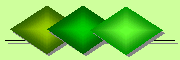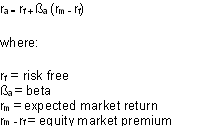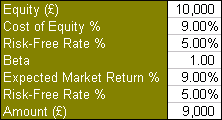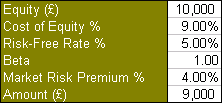De Claron LtdExpress Accountancy services at affordable prices
 Accounting Property Investment Reporting Company Secretarial ServicesHelp

Capital Asset Pricing Model (CAPM)

Capital Asset Pricing Model (CAPM) for an investment property defines the relationship between the risk of investing in the property and the return which that property is expected to generate for the investor. The CAPM is based on the notion that the investor is to be compensated for:

• time value of money for a risk free investment
• risk value of money if invested elsewhere
• These two components are represented in the formula:The time value of money is represented by the risk-free (rf) rate in the formula and compensates the investors for placing money in any investment property over a period of time.

The risk value is represented by the other half of the formula which calculates the amount of compensation the investor needs for taking on additional risk. This is calculated by taking a risk measure (beta) that compares the returns of the investment property to the market over a period of time and to the market premium (rm-rf). Note that beta is also referred to as systematic risk or market risk.

For example, a company acquires an investment property for \$100,000 which is expected to generate rental income. If the same money was put in government bonds the amount of guaranteed or risk free income is 5% per annum. The volatility of the rental market for investment properties is expressed as a beta value of 1.0. Using the above formula the risk value or cost of equity of the property is 9% per annum which equates to \$9,000 expected annual return income This is almost twice the 5% return obtained from government bonds making the investment worthwhile.Note that the above formula can be condensed by denoting (rm-rf) as Market Risk Premium:

• Cost of Equity = Risk-Free Rate + (Beta x Market Risk Premium).
•Related topics:

• Cost of Capital
• Discounted Cash Flows